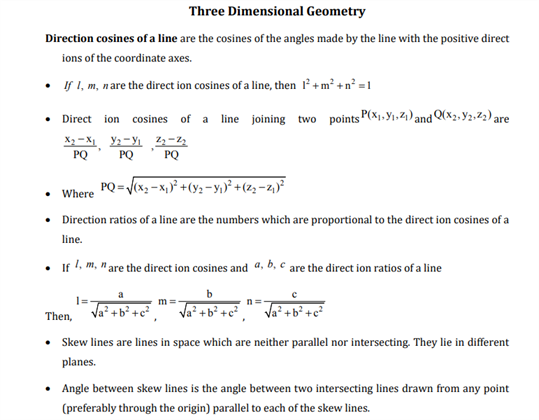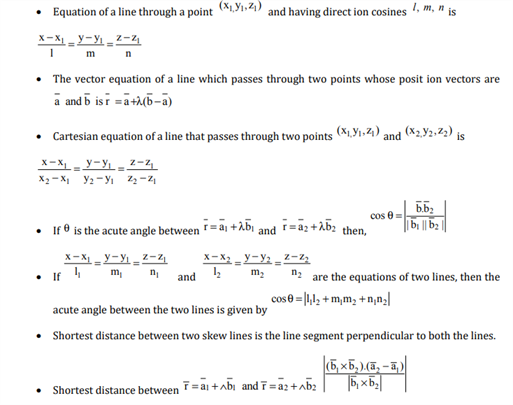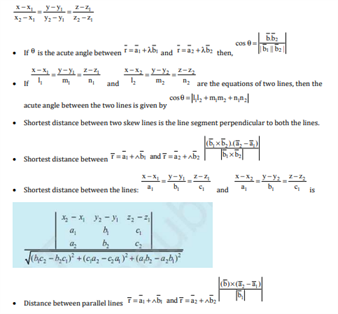# Maths Revision Notes for Class 12 Chapter 11 Three Dimensional Geometry.

Three Dimensional Geometry is the 11th chapter present in the CBSE Class 12 Maths Revision Notes. It can be defined as the mathematical interpretation of 3D shapes such as tetrahedrons, cuboids, and cubes. The major point that differentiates two dimensional and three dimensional geometrical objects lies simply in the fact, that three-dimensional objects have height, width and depth. Some of the other concepts that are introduced in this chapter are:

• The Central Idea of 3D
• Axes
• Coordinate Planes
• Coordinate of a point
• Vector representation of a point in space
• Distance formula
• Distance of a point P from coordinate axes
• Section formula
• Centroid of a Tetrahedron
• Relation between two lines
• Direction Cosines and Direction Ratios
• Angle between two line segments
• Projection of a line segment on a line
• Equation of a Plane
• Angle between two planes

You can find the revision notes for chapter 11, available below:You may also want to read related articles like: